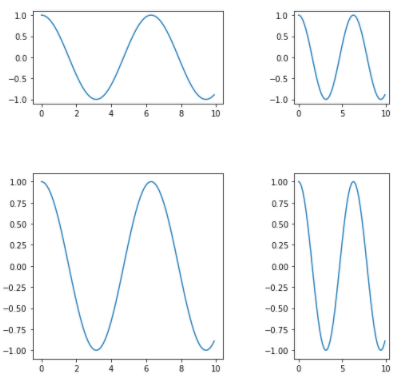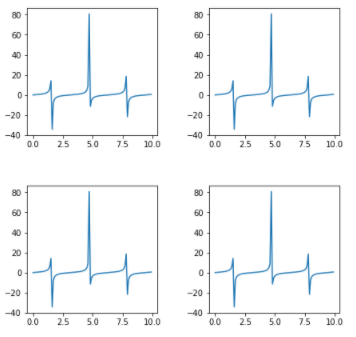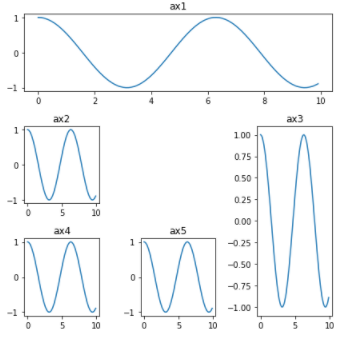Related Articles

# How to Create Different Subplot Sizes in Matplotlib?

• Last Updated : 23 Sep, 2021

In this article, we will learn different ways to create subplots of different sizes using Matplotlib. It provides 3 different methods using which we can create different subplot of different sizes.

Methods available to create subplot :

• Gridspec
• gridspec_kw
• subplot2grid

1. Gridspec : GridSpec from the gridspec module used to adjust the geometry of Subplot grid. We can use different parameters to adjust shape, size, no. of columns and rows.

Code :

## Python3

 `# importing required libraries` `import` `matplotlib.pyplot as plt` `from` `matplotlib ``import` `gridspec` `import` `numpy as np`   `# create a figure` `fig ``=` `plt.figure()`   `# to change size of subplot's` `# set height of each subplot as 8` `fig.set_figheight(``8``)`   `# set width of each subplot as 8` `fig.set_figwidth(``8``)`   `# create grid for different subplots` `spec ``=` `gridspec.GridSpec(ncols``=``2``, nrows``=``2``,` `                         ``width_ratios``=``[``2``, ``1``], wspace``=``0.5``,` `                         ``hspace``=``0.5``, height_ratios``=``[``1``, ``2``])`   `# initializing x,y axis value` `x ``=` `np.arange(``0``, ``10``, ``0.1``)` `y ``=` `np.cos(x)`   `# ax0 will take 0th position in ` `# geometry(Grid we created for subplots),` `# as we defined the position as "spec"` `ax0 ``=` `fig.add_subplot(spec[``0``])` `ax0.plot(x, y)`   `# ax1 will take 0th position in` `# geometry(Grid we created for subplots),` `# as we defined the position as "spec"` `ax1 ``=` `fig.add_subplot(spec[``1``])` `ax1.plot(x, y)`   `# ax2 will take 0th position in` `# geometry(Grid we created for subplots),` `# as we defined the position as "spec"` `ax2 ``=` `fig.add_subplot(spec[``2``])` `ax2.plot(x, y)`   `# ax3 will take 0th position in` `# geometry(Grid we created for subplots),` `# as we defined the position as "spec"` `ax3 ``=` `fig.add_subplot(spec[``3``])` `ax3.plot(x, y)`   `# display the plots` `plt.show()`

Output :Explanation :

# create grid for different subplots
spec = gridspec.GridSpec(ncols=2, nrows=2, width_ratios=[2, 1],wspace=0.5,hspace=0.5,height_ratios=[1,2])

Here “gridspec.GridSpec()” will create grids for subplots.We can use different parameters to adjust the grid and each plot size.

• ncols : pass number of columns you want in Grid.
• nrows : pass number of rows we want in Grid to make subplots.
• width_ratios : set width ratio of subplot(adjust the width of plot).
• height_ratios : set height ratio of subplot(adjust the height of plot).
• wspace : give “wspace” amount of space vertically to separate the subplots.
• hspace : give “hspace” amount of space horizontally to separate the subplots.

2. gridspec_kw : It is a dictionary available inside “plt.subplots()” method in Matplotlib. By passing different parameters to the dictionary we can adjust the shape and size of each subplot.

Code :

## Python3

 `# importing required libraries` `import` `matplotlib.pyplot as plt` `import` `numpy as np`   `# setting different parameters to adjust each grid` `fig, ax ``=` `plt.subplots(nrows``=``2``, ncols``=``2``, figsize``=``(``7``, ``7``),` `                       ``gridspec_kw``=``{` `                           ``'width_ratios'``: [``3``, ``3``],` `                           ``'height_ratios'``: [``3``, ``3``],` `                       ``'wspace'``: ``0.4``,` `                       ``'hspace'``: ``0.4``})`     `# initializing x,y axis value` `x ``=` `np.arange(``0``, ``10``, ``0.1``)` `y ``=` `np.tan(x)`   `# ax will take 0th position in ` `# geometry(Grid we created for subplots)` `ax[``0``][``0``].plot(x, y)`   `# ax will take 0th position in` `# geometry(Grid we created for subplots)` `ax[``0``][``1``].plot(x, y)`   `# ax will take 0th position in` `# geometry(Grid we created for subplots)` `ax[``1``][``0``].plot(x, y)`   `# ax will take 0th position in` `# geometry(Grid we created for subplots)` `ax[``1``][``1``].plot(x, y)`   `plt.show()`

Output :Explanation :

# setting different parameters to adjust each grid
fig, ax = plt.subplots(nrows=2, ncols=2,figsize=(7,7), gridspec_kw={‘width_ratios’: [3, 3], ‘height_ratios’: [3, 3], ‘wspace’ : 0.4, ‘hspace’ : 0.4})

“gridspec_kw = {}” is dictionary with keywards using which we can change shape, size and adjust each grid.

• nrows : number of rows in grid
• ncols : number of columns in grid
• width_ratios : set width size of each subplot
• height_ratios : set height size of each subplot
• wspace : give “wspace” amount of space vertically to separate the subplots.
• hspace : give “hspace” amount of space horizontally to separate the subplots.
• figsize : set size of subplot’s.

3. subplot2grid : It provides more flexibility to create a grid at any location. We can extend the grid horizontally as well as vertically very easily.

Code :

## Python3

 `# importing required library` `import` `matplotlib.pyplot as plt` `import` `numpy as np`   `# creating grid for subplots` `fig ``=` `plt.figure()` `fig.set_figheight(``6``)` `fig.set_figwidth(``6``)`   `ax1 ``=` `plt.subplot2grid(shape``=``(``3``, ``3``), loc``=``(``0``, ``0``), colspan``=``3``)` `ax2 ``=` `plt.subplot2grid(shape``=``(``3``, ``3``), loc``=``(``1``, ``0``), colspan``=``1``)` `ax3 ``=` `plt.subplot2grid(shape``=``(``3``, ``3``), loc``=``(``1``, ``2``), rowspan``=``2``)` `ax4 ``=` `plt.subplot2grid((``3``, ``3``), (``2``, ``0``))` `ax5 ``=` `plt.subplot2grid((``3``, ``3``), (``2``, ``1``), colspan``=``1``)`     `# initializing x,y axis value` `x ``=` `np.arange(``0``, ``10``, ``0.1``)` `y ``=` `np.cos(x)`   `# plotting subplots` `ax1.plot(x, y)` `ax1.set_title(``'ax1'``)` `ax2.plot(x, y)` `ax2.set_title(``'ax2'``)` `ax3.plot(x, y)` `ax3.set_title(``'ax3'``)` `ax4.plot(x, y)` `ax4.set_title(``'ax4'``)` `ax5.plot(x, y)` `ax5.set_title(``'ax5'``)`   `# automatically adjust padding horizontally ` `# as well as vertically.` `plt.tight_layout()`   `# display plot` `plt.show()`

Output :Explanation :

ax1 = plt.subplot2grid(shape=(3, 3), loc=(0, 0), colspan=3)
ax2 = plt.subplot2grid(shape=(3, 3), loc=(1, 0), colspan=1)
ax3 = plt.subplot2grid(shape=(3, 3), loc=(1, 2), rowspan=2)
ax4 = plt.subplot2grid((3, 3), (2, 0))
ax5 = plt.subplot2grid((3, 3), (2, 1),colspan=1)

Here shape is denoting to no. of rows and columns and loc denotes to geometric location of grid. Suppose if we are using colspan = 3 in ax1,it means these subplot will cover 3 all columns of that row. Similarly in ax2,colspan=1 means it will cover 1 column space at its location. In case of ax3,rowspan=2 means it will cover space of 2 rows.If we give irregular input then it will give error so we have give proper value to colspan and rowspan.

Attention geek! Strengthen your foundations with the Python Programming Foundation Course and learn the basics.

To begin with, your interview preparations Enhance your Data Structures concepts with the Python DS Course. And to begin with your Machine Learning Journey, join the Machine Learning – Basic Level Course

My Personal Notes arrow_drop_up
Recommended Articles
Page :